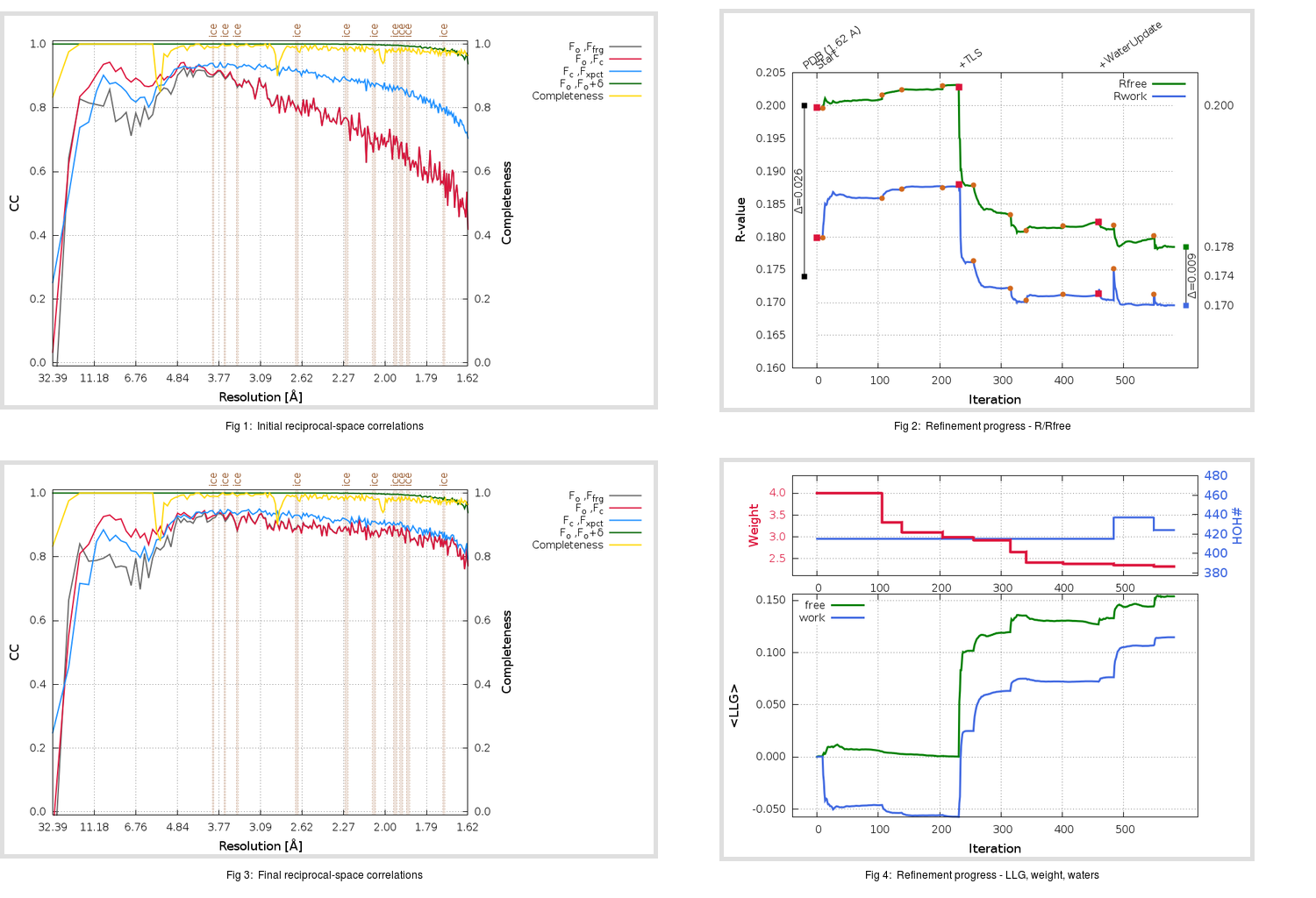Content:

## Deposited

` `
 Date deposited Date data collection Resolution R, Rfree 20170205 20121201 1.62 0.1720 0.2000

Molprobity (CCP4 7.0 version) summary:

```Ramachandran outliers =   0.00 %
favored =  98.36 %
Rotamer outliers      =   1.50 %
C-beta deviations     =     5
Clashscore            =   3.34
RMS(bonds)            =   0.0283
RMS(angles)           =   2.43
MolProbity score      =   1.26
Resolution            =   1.62
R-work                =   0.1720
R-free                =   0.2000
```

```Number of waters      =   415

<B> (all atoms) =   34.98 ( sd =   11.52 ) for       2852 non-hydrogen atoms
<B>   (protein) =   32.66 ( sd =   10.18 ) for       2391 non-hydrogen atoms
<B>     (water) =   46.90 ( sd =   10.03 ) for        415 non-hydrogen atoms
<B>    (others) =   46.32 ( sd =   13.90 ) for         46 non-hydrogen atoms

B min/max       (all non-hydrogen atoms) =   18.99 /  103.46
B min/max   (protein non-hydrogen atoms) =   18.99 /  103.46
B min/max     (water non-hydrogen atoms) =   22.29 /   72.75
B min/max     (other non-hydrogen atoms) =   28.05 /   79.59
```

## BUSTER (re-)refinement

` `

Molprobity (CCP4 7.0 version) summary:

```Ramachandran outliers =   0.00 %
favored =  97.70 %
Rotamer outliers      =   1.87 %
C-beta deviations     =     0
Clashscore            =   1.67
RMS(bonds)            =   0.0119
RMS(angles)           =   1.54
MolProbity score      =   1.19
Resolution            =   1.62
R-work                =   0.1696
R-free                =   0.1785
```

```Number of waters      =   424

<B> (all atoms) =   34.80 ( sd =   11.13 ) for       2861 non-hydrogen atoms
<B>   (protein) =   31.94 ( sd =    8.07 ) for       2391 non-hydrogen atoms
<B>     (water) =   49.96 ( sd =   12.44 ) for        424 non-hydrogen atoms
<B>    (others) =   42.58 ( sd =   14.51 ) for         46 non-hydrogen atoms

B min/max       (all non-hydrogen atoms) =   18.84 /   95.32
B min/max   (protein non-hydrogen atoms) =   18.84 /   63.43
B min/max     (water non-hydrogen atoms) =   21.96 /   95.32
B min/max     (other non-hydrogen atoms) =   29.40 /   86.50
```

Refinement progression:Results:

` `
 File Remark 5N19_aB_refine.01_03_refine.pdb.gz exact refinement commands are in header 5N19_aB_refine.01_03_refine.mtz.gz including original deposited data and several re-refinement map coefficients 5N19_aB_refine.01_03_BUSTER_model.cif.gz including any non-standard compound restraints 5N19_aB_refine.01_03_BUSTER_refln.cif.gz